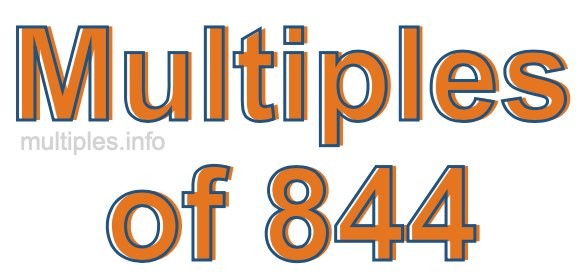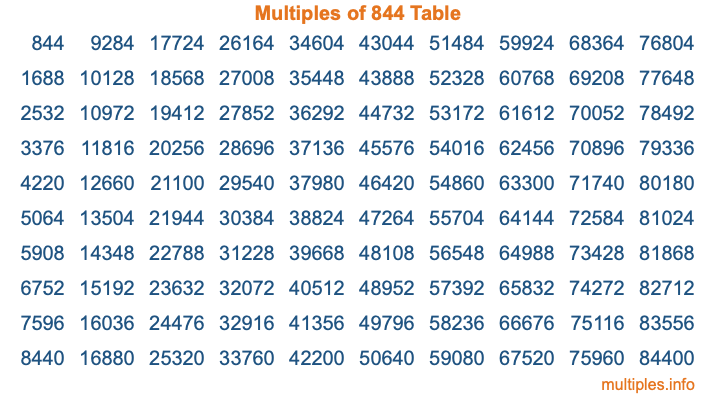Multiples of 844Welcome to the Multiples of 844 page. Here we will first teach you everything you will ever need to know about the multiples of 844, and then give you a study guide summary of everything we taught you to make sure you remember it all. Use this page to look up facts and learn information about the multiples of 844. This page will make you a multiples of eight hundred forty-four expert!

Definition of Multiples of 844
Multiples of 844 are all the numbers that when divided by 844 equal an integer. Each of the multiples of 844 are called a multiple. A multiple of 844 is created by multiplying 844 by an integer.

Therefore, to create a list of multiples of 844, you start with 1 multiplied by 844, then 2 multiplied by 844, then 3 multiplied by 844, and so on for as long as you want. Thus, the list of the first five multiples of 844 is 844, 1688, 2532, 3376, and 4220. To see a larger list of multiples of 844, see the printable image of Multiples of 844 further down on this page. We also have a category where you can choose any nth multiple of 844.

Multiples of 844 Checker
The Multiples of 844 Checker below checks to see if any number of your choice is a multiple of 844. In other words, it checks to see if there is any number (integer) that when multiplied by 844 will equal your number. To do that, we divide your number by 844. If the the quotient is an integer, then your number is a multiple of 844.

Is  a multiple of 844?

Least Common Multiple of 844 and ...
A Least Common Multiple (LCM) is the lowest multiple that two or more numbers have in common. This is also called the smallest common multiple or lowest common multiple and is useful to know when you are adding our subtracting fractions. Enter one or more numbers below (844 is already entered) to find the LCM.

Check out our LCM Calculator if you need more details about the Least Common Multiple or if you need the LCM for different numbers for adding and subtraction fractions.

nth Multiple of 844
As we stated above, 844 is the first multiple of 844, 1688 is the second multiple of 844, 2532 is the third multiple of 844, and so on. Enter a number below to find the nth multiple of 844.

th multiple of 844

Multiples of 844 vs Factors of 844
844 is a multiple of 844 and a factor of 844, but that is where the similarities end. All postive multiples of 844 are 844 or greater than 844. All positive factors of 844 are 844 or less than 844.

Below is the beginning list of multiples of 844 and the factors of 844 so you can compare:

Multiples of 844: 844, 1688, 2532, 3376, 4220, etc.

Factors of 844: 1, 2, 4, 211, 422, 844

As you can see, the multiples of 844 are all the numbers that you can divide by 844 to get a whole number. The factors of 844, on the other hand, are all the whole numbers that you can multiply by another whole number to get 844.

It's also interesting to note that if a number (x) is a factor of 844, then 844 will also be a multiple of that number (x).

Multiples of 844 vs Divisors of 844
The divisors of 844 are all the integers that 844 can be divided by evenly. Below is a list of the divisors of 844.

Divisors of 844: 1, 2, 4, 211, 422, 844

The interesting thing to note here is that if you take any multiple of 844 and divide it by a divisor of 844, you will see that the quotient is an integer.

Multiples of 844 Table
Below is an image of the first 100 multiples of 844 in a table. The table is in chronological order, column by column. The first column has the first ten multiples of 844, the second column has the next ten multiples of 844, and so on.The Multiples of 844 Table is also referred to as the 844 Times Table or Times Table of 844. You are welcome to print out our table for your studies.

Negative Multiples of 844
Although not often discussed or needed in math, it is worth mentioning that you can make a list of negative multiples of 844 by multiplying 844 by -1, then by -2, then by -3, and so on, to get the following list of negative multiples of 844:

-844, -1688, -2532, -3376, -4220, etc.

Multiples of 844 Summary
Below is a summary of important Multiples of 844 facts that we have discussed on this page. To retain the knowledge on this page, we recommend that you read through the summary and explain to yourself or a study partner why they hold true.

There are an infinite number of multiples of 844.

A multiple of 844 divided by 844 will equal a whole number.

844 divided by a factor of 844 equals a divisor of 844.

The nth multiple of 844 is n times 844.

The largest factor of 844 is equal to the first positive multiple of 844.

844 is a multiple of every factor of 844.

844 is a multiple of 844.

A multiple of 844 divided by a divisor of 844 equals an integer.

844 divided by a divisor of 844 equals a factor of 844.

Any integer times 844 will equal a multiple of 844.

Multiples of a Number
Here you can get the multiples of another number, all with the same attention to detail as we did for multiples of 844 on this page.

Multiples of
Multiples of 845
Did you find our page about multiples of eight hundred forty-four educational? Do you want more knowledge? Check out the multiples of the next number on our list!